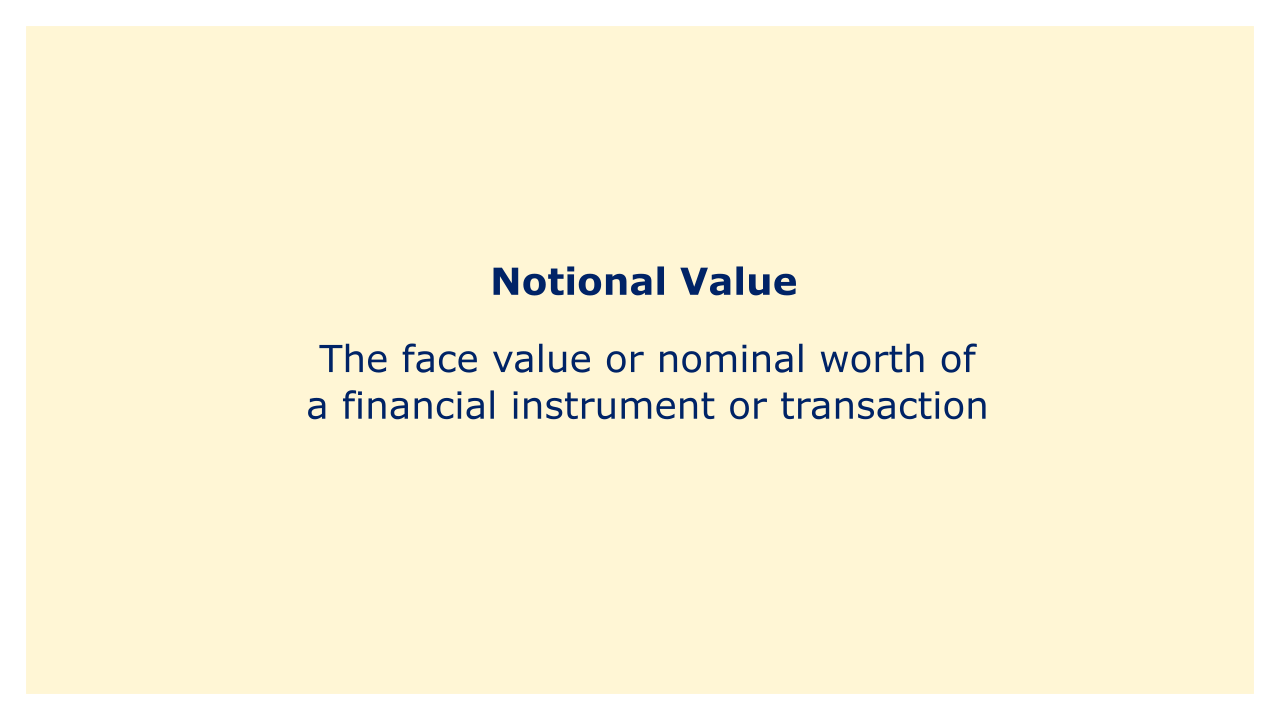# Notional ValueImage: Moneybestpal.com

### The word notional value is used in finance to describe the face value or nominal worth of a financial instrument or transaction. It is a hypothetical value that is used to determine obligations and payments, but it does not always correspond to the actual value of the instrument or transaction.

Options, futures, and swap contracts are examples of derivatives that frequently use the notional value. In these kinds of contracts, the underlying asset on which the contract is based is represented by the notional value. For instance, if the price of oil is \$100 per barrel, the notional value of a futures contract on oil might be \$100,000, or the value of 1,000 barrels of oil.

To determine how much will be paid or owed under the contract, the notional value is employed. The notional value, for instance, in a swap contract, is the quantity of the underlying asset that is being traded between the parties. The payments under the swap contract are normally calculated based on the discrepancy between the notional value and the actual value of the underlying asset at the time the payment is due.

The notional value does not always correspond to the true worth of the underlying item or the transaction, it is vital to remember this. For instance, a swap contract's notional value might be far higher than the real value of the underlying assets being traded, and the payments paid under the contract might be determined by changes in the assets' value rather than the notional value.

Notional value can be utilized in financial transactions other than derivatives contracts, including loans and bonds. In these situations, the principal amount of the loan or bond is represented by the notional value, which is utilized to determine interest payments and other obligations.
Tags